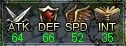## FANDOM

608 PagesAttributes

The are four principal attributes: ATK, DEF, SPD, and INT each of which affect Heroes in very important ways.

## Reference EquationsEdit

The equations set out below are part of a system of equations that govern Combat Mechanics, and are provided here for reference. Base unit damage is the damage that one unit will do to another unit absent any modifiers from equipment or Hero stats. The Hero stats equation describes how Hero stats affect base unit damage.

Base unit damage: $\frac{{\text{ATK}_1}^2}{\text{ATK}_1+\text{DEF}_2}$

• $\text{ATK}_1$ is the attacking unit's ATK stat.
• $\text{DEF}_2$ is the defending unit's DEF stat.

Hero Stats equation: $\frac{1+\text{HATK}_1/100}{1+\text{HDEF}_2 / 100}$

• $\text{HATK}_1$ is the attacking Hero's attack score.
• $\text{HDEF}_2$ is the defending Hero's defense score.
• Note: This equation typically produces a decimal number between 0.33 and 3.00 which modifies base unit damage.

## ATKEdit

HATK increases the attack of a Hero's units according to base unit damage * ($1 + \text{HATK}_1 / 100$). Thus, each point of ATK will raise a unit's base unit damage damage by 1%, assuming that $\text{HDEF}_2$ = 0.

Example

Hero has 50 ATK. (1 + 50/100) / (1 + 0/100) = 1.5. This 1.5 bonus will raise base unit damage by 50%. This will vary depending on which units are attacking and defending.

• Note: This example is only true versus NPCs (which have HDEF of' 0), as a Hero controlled by a human player will never have 0 HDEF.

## DEFEdit

HDEF decreases the attack of a Hero's units according to: base unit damage * $\frac{1}{1 + \text{HDEF}_2 / 100}$.

Example

Hero has 50 DEF. 1 / (1 + 50/100) = 1/1.5 = $0.\overline{6}$. Thus, one would multiply base unit damage * $0.\overline{6}$.

• This assumes that the opposing Hero's HATK is 0, which will never be the case against a Human or NPC opponent. Also notice that HDEF is deceptive: it doesn't actually affect the defense score of the units being attacked, but rather serves as part of a ratio that modifies damage done by attacking units.
• Against NPC's, the opposing invisible Hero's HATK is 10, so therefore the correct equation against NPC's without a Hero is $\frac{1.1}{1 + \text{HDEF}_2 / 100}$, or $1.1/1.5 = 0.7\overline{3}$ in the previous case.

## SPDEdit

SPD has several beneficial effects:

• First, SPD increases Hero movements speed on the world map. The mechanism is currently unknown.
• Second, SPD raises unit speed according to the equation (HSPD -10) * 0.02.
Example

Tier 8 units all have 7.2 SPD. If you want to beat a Elf Dragon NPC, which has 10.11 SPD, do the following calculations:

10.11 - 7.2 = 2.91 additional SPD required to be faster than Elf Dragon

How many SPD attribute points required (including bonuses)?

(HSPD - 10) * 0.02 = 2.91 (divide both sides by 0.02).
HSPD - 10 = 145.5 (round up to 146 to be safe).
HSPD = 146 + 10 = 156 SPD required for Tier 8 units to take priority over NPC Elf Dragon.
• Finally, SPD governs how far melee units will travel on the battlefield. For this equation, use (HSPD -10) * 0.02 + Unit SPD * (1+ %modifiers).
• 10 SPD required for front row units to attack other front row units. Elf Dragon can do this naturally.
• 12 SPD required for front row units to attack back row units.
• 14 SPD required for back row units to attack back row units.

Going by the above equation, you need the following speed for the different races T10 units to reach the back row from your front row (with no modifier):

• Human - 116 (will show maths for first one: 116-10= 106. 106*0.02 = 2.12. 2.12 + 9.89 = 12.01)
• Elf - 105
• Undead - 116
• Orc - 134

Using the above numbers, add 100 to work out how much you need to get from your back row to their back row. As 100*0.02 = 2, and the difference between one row and the next is 2. Notice: Updated (mostly removed false information) as the developers released the actual formula to us at gPotato's server.

## INTEdit

One of the effects of INT is namely that it increases hourly gold output of the Townhall.

• Each point of INT above 10 raises a Castle's hourly Gold output by $0.\overline{6}$% of basic Townhall gold output.
• Example: Castellan has 50 INT; Townhall has 825 hourly output. New gold output is (50 - 10) * $0.\overline{6}$ * 825/100.
• Thus, 40 * $0.\overline{6}$ * 8.25 = 220

Note: This number does not account for additional resource production bonuses. For further information, see Castellan.

INT affects the damage done by scrolls:

• A Hero's INT stat affects spell damage according to the following formula: base spell damage * (1 + INT/50).
• A Hero's INT stat might also affect spell damage received from an enemy who is using scrolls.

## NPC Invisible Hero AttributesEdit

All NPC units have an invisible "Hero" that grants nominal combat bonuses:

• 10 ATK
• 0 DEF
• 10 SPD

Notice: this is not actual a hidden NPC, but is part of the calculate formula, rest of the page needs to be updated (when someone has time, already did SPD and someone else did DEF). The effect just isn't noticeable on PvP because it affects both sides.